# The LOAN Procedure

### Computational Details

These terms are used in the formulas that follow:

p

periodic payment

a

principal amount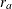nominal annual rate

f

compounding frequency (per year)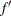payment frequency (per year)

r

periodic rate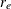effective interest rate

n

total number of payments

The periodic rate, or the simple interest applied during a payment period, is given by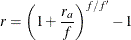Note that the interest calculation is performed at each payment period rather than at the compound period. This is done by adjusting the nominal rate. See Muksian (1984) for details.

Note that when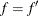(that is, when the payment and compounding frequency coincide), the preceding expression reduces to the familiar form: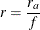The periodic rate for continuous compounding can be obtained from this general expression by taking the limit as the compounding frequency f goes to infinity. The resulting expression is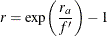The effective interest rate, or annualized percentage rate (APR), is that rate which, if compounded once per year, is equivalent to the nominal annual rate compounded f times per year. Thus,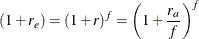or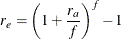For continuous compounding, the effective interest rate is given by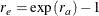See Muksian (1984) for details.

The payment is calculated as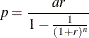The amount is calculated as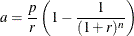Both the payment and amount are rounded to the nearest hundredth (cent) unless the ROUND= specification is different than the default, 2.

The total number of payments n is calculated as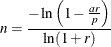The total number of payments is rounded up to the nearest integer.

The nominal annual rate is calculated using the bisection method, with a as the objective and r starting in the interval between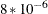and 0.1 with an initial midpoint 0.01 and successive midpoints bisecting.